# AP Chemistry : Thermodynamics

## Example Questions

### Example Question #3 : Hess' Law

Using the following data to calculate the enthalpy change in:  C4H10O(l)  ->  C4H8 (g)  +  H2O (g)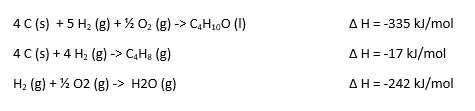76 kJ/mol

-76 kJ/mol

152 kJ/mol

-17 kJ/mol

-152 kJ/mol

76 kJ/mol

Explanation: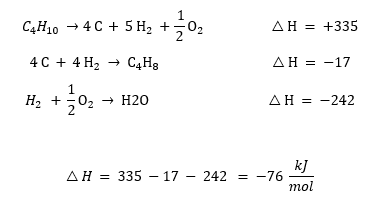### Example Question #4 : Hess' Law

Using the following data to calculate the enthalpy change in:  CaO (s) + H2O (l)  ->  Ca(OH)2 (aq)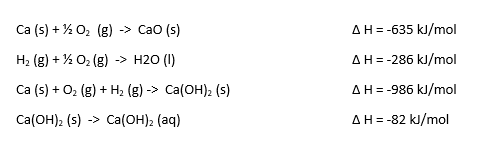-62 kJ/mol

-124 kJ/mol

-147 kJ/mol

124 kJ/mol

147 kJ/mol

-147 kJ/mol

Explanation: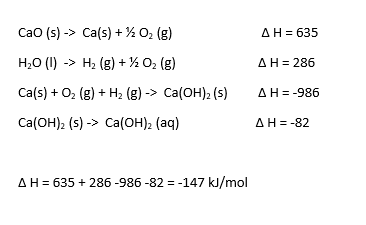### Example Question #5 : Hess' Law

Using the following data to calculate the enthalpy change in:  B2H6 (g) + 3 O2 (g) -> B2O3 (s) + 3 H2O (l)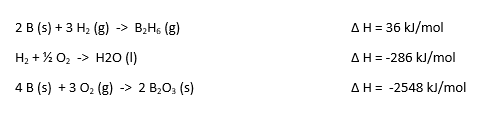2168 kJ/mol

1084 kJ/mol

3252 kJ/mol

-2168 kJ/mol

-1084 kJ/mol

-2168 kJ/mol

Explanation: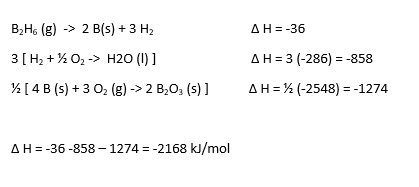### Example Question #1 : Cell Potential Under Non Standard Conditions

Consider an electrochemical cell that has the following overall reaction:

2 H+(aq) + Sn (s) -> Sn2+ (aq) + H2 (aq)

Which of the following changes would alter the measured cell potential?

Lowering the pH in the cathode.

All of the above.

Increase the pH in the cathode.

Increasing the H2 pressure in the cathode.

All of the above.

Explanation: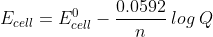All of these changes would change Q and thus change the measured cell potential.

### Example Question #2 : Cell Potential Under Non Standard Conditions

For the following cell reaction:

2 Al (s) + 3 Mn2+ (aq) -> 2 Al3+ (aq)  + 3 Mn (s)

predict if the cell potential Ecell will be larger, or smaller than Eocell for the following conditions:

[Al3+] = 2.0 M; [Mn2+] = 1.0 M.

Identical

Smaller

Larger

Can not be determined

Smaller

Explanation:Altering these conditions would increase Q, and thus result in a decrease in the measured cell potential.

### Example Question #3 : Cell Potential Under Non Standard Conditions

For the following cell reaction:

2 Al (s) + 3 Mn2+ (aq) -> 2 Al3+ (aq)  + 3 Mn (s)

predict if the cell potential Ecell will be larger, or smaller than Eocell for the following conditions:  [Al3+] = 1.0 M; [Mn2+] = 5.0 M.

Identical

Can not be determined

Larger

Smaller

Larger

Explanation:At these concentrations Q will become smaller, and thus log Q will become smaller.  This will give rise to a larger cell potential.

### Example Question #4 : Cell Potential Under Non Standard Conditions

What is the cell potential of the following cell:

Zn (s) + 2 H+(aq)  ->  Zn2+ (aq)  +  H2 (g)                                  Eo = 0.76 V

When the [Zn2+] = 1.0 M; PH2 = 1 atm, and the pH in the cathode is 5.2?

1.80 V

1.59 V

0.45 V

1.32 V

0.90 V

0.45 V

Explanation: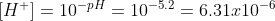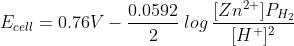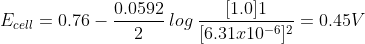### Example Question #5 : Cell Potential Under Non Standard Conditions

Calculate the standard cell potential of the following reaction:

Cd(s) +  MnO2 (s) + 4 H+ (aq) +  ->  Cd2+ (aq) +  Mn2+ (aq)  + 2 H2O (l)

Given:

MnO2 (s) + 4 H+ (aq)  + 2e-  ->  Mn2+ (aq) + 2 H2O (l)            Eo = 1.23 V

Cd2+ (aq)  + 2 e-   ->  Cd (s)                                                     Eo = -0.40 V

1.63 V

0.0 V

0.83 V

-1.63 V

-0.83 V

1.63 V

Explanation:

Eocell  = Eo cathode - Eoanode

Eocell = 1.23 – (-0.40) = 1.63 V

### Example Question #6 : Cell Potential Under Non Standard Conditions

Calculate the standard cell potential of the following reaction:

Zn (s) + Cu2+ (aq)  ->  Zn2+ (aq) + Cu (s)

Given:

Zn2+(aq)+ 2 e-->  Zn (s)                                             Eo = -0.76 V

Cu2+(aq)+ 2 e-->  Cu (s)                                             Eo = 0.34 V

-0.42 V

0.0 V

1.10 V

-1.10 V

0.42 V

1.10 V

Explanation:

Eocell  = Eo cathode - Eoanode

Eocell = 0.34 – (-0.76) = 1.10 V

### Example Question #7 : Cell Potential Under Non Standard Conditions

Calculate the standard cell potential of the following reaction:

Zn (s) + 2 Ag1+ (aq)  ->  Zn2+ (aq) + 2 Ag (s)

Given:

Zn2+(aq)+ 2 e-->  Zn (s)                                             Eo = -0.76 V

Ag1+(aq)+ 1 e-->  Ag (s)                                             Eo = 0.80 V

2.36 V

-0.04 V

-1.56 V

1.56 V

0.04 V